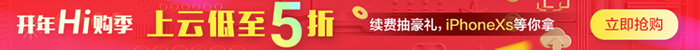#poj 1477 Box of Bricks

1. 云栖社区>
2. 博客>
3. 正文## poj 1477 Box of Bricks

this_is_bill 2013-10-31 11:02:00 浏览687 评论0

AC的代码：

```#include <iostream>

inline int Abs(int n){return n<0?(-n):n;}

int main()
{
int count=0;
int n,i;
int a;
int sum;
int result;
//n!=0 等价于 n
while(scanf("%d",&n) && n)
{
count++;
printf("Set #%d\n",count);

sum=0;
for(i=1;i<=n;i++)
{
scanf("%d",&a[i]);
sum+=a[i];
}

sum/=n;
result=0;
for(i=1;i<=n;i++)
result+=Abs(sum-a[i]);

printf("The minimum number of moves is %d.\n\n",result/2);
}

return 0;
}```

【云栖快讯】一站式开发者服务，海量学习资源免费学  详情请点击

this_is_bill

+ 关注